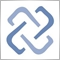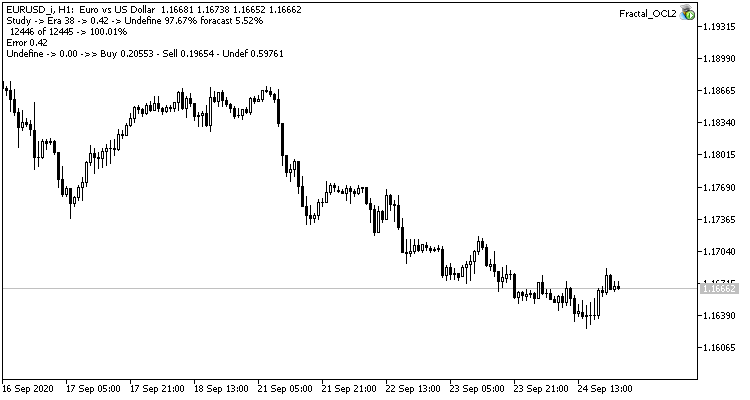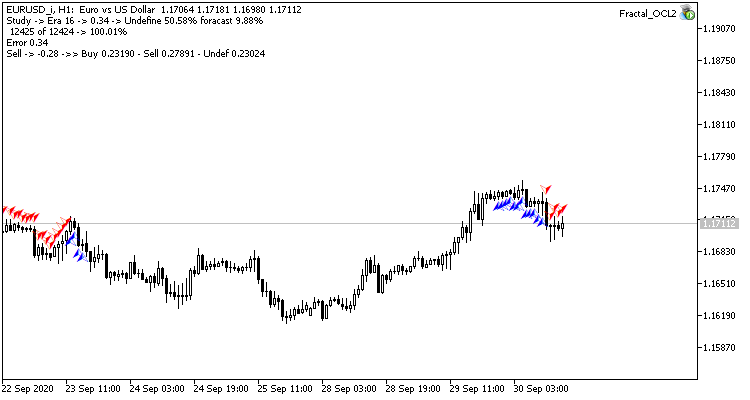# Discussion of article "Neural networks made easy (Part 6): Experimenting with the neural network learning rate"Moderator
228934

New article Neural networks made easy (Part 6): Experimenting with the neural network learning rate has been published:

We have previously considered various types of neural networks along with their implementations. In all cases, the neural networks were trained using the gradient decent method, for which we need to choose a learning rate. In this article, I want to show the importance of a correctly selected rate and its impact on the neural network training, using examples.

The third experiment is a slight deviation from the main topic of the article. Its idea came about during the first two experiments. So, I decided to share it with you. While observing the neural network training, I noticed that the probability of the absence of a fractal fluctuates around 60-70% and rarely falls below 50%. The probability of emergence of a fractal, wither buy or sell, is around 20-30%. This is quite natural, as there are much less fractals on the chart than there are candlesticks inside trends. Thus, our neural network is overtrained, and we obtain the above results. Almost 100% of fractals are missed, and only rare ones can be caught.To solve this problem, I decided to slightly compensate for the unevenness of the sample: for the absence of a fractal in the reference value, I specified 0.5 instead of 1 when training the network.

```            TempData.Add((double)buy);
```

This step produced a good effect. The Expert Advisor running with a learning rate of 0.01 and a weight matrix obtained from previous experiments shows the error stabilization of about 0.34 after 5 training epochs. The share of missed fractals decreased to 51% and the percentage of hits increased to 9.88%. You can see from the chart that the EA generates signals in group and thus shows some certain zones. Obviously, the idea requires additional development and testing. But the results suggest that this approach is quite promising.Author: Dmitriy Gizlyk25

Hi Dmitriy,

I really like this series, as a learning tool for me for Neural Networks. I use MT4, including finding an implementation of SymbolInfo. I am guess that is where the problem is, as it is running but not doing anything during learning. Would you have any idea on what would be needed for it to run in MT4? Thanks!